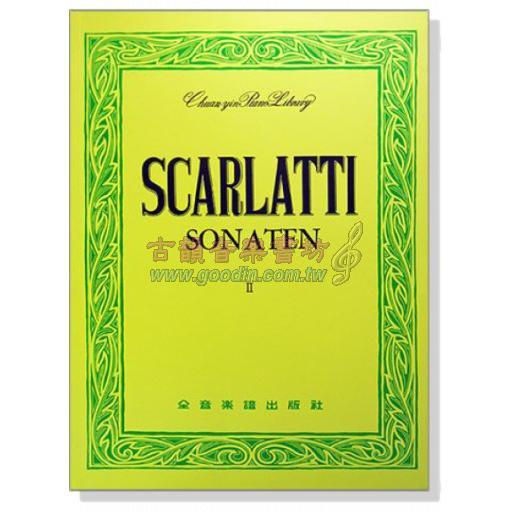# 史卡拉第 奏鳴曲集【II】---世界音樂全集9## 商品標籤

史卡拉第作品充分流露出大鍵琴的韻味。並以奏鳴曲獨特的曲式、風格與和聲，來闡明在奏鳴曲發展史上承先啟後的地位。第二冊由簡至難，以調性排列收錄後25首著名的奏鳴曲作品。

Scarlatti Sonaten Ⅱ Song List:

L.1(K. 514) , L.8(K. 461) , L.10(K. 84) , L.17(K. 371) , L.20(K. 51) , L.23(K. 380) , L.25(K. 46) , L.35(K. 319) , L.43(K. 405) , L.58(K. 64) , L.98(K. 373) ,L.104(K. 159) , L.107(K. 140) , L.175(K. 387) , L.186(K. 127) , L.188(K. 525) , L.231(K. 31) ,L.234(K. 390) , L.260(K. 246) , L.279(K. 419) , L.282(K. 133) , L.283(K. 524) , L.293(K. 537) , L.301(K. 49) ,L.304(K. 470) , L.307(K. 269) , L.340(K. 476) , L.348(K. 244) , L.349(K. 146) , L.366(K. 1) , L.369(K. 145) , L.374(K. 15) ,L.378(K. 3) , L.380(K. 203) , L.381(K. 438) , L.395(K. 533) , L.397(K. 16) , L.402(K. 126) , L.411(K. 23) , L.414(K. 388) ,L.424(K. 33) , L.442(K. 104) , L.443(K. 356) , L.447(K. 173) , L.469(K. 110) , L.473(K. 183) , L.481(K. 25) , L.487(K. 125) , L.489(K. 12)

### 購買過此商品的人還購買過